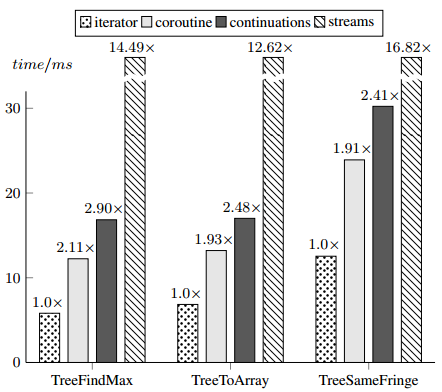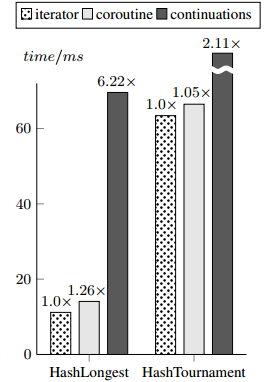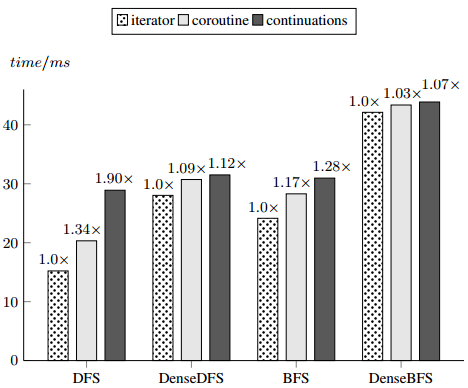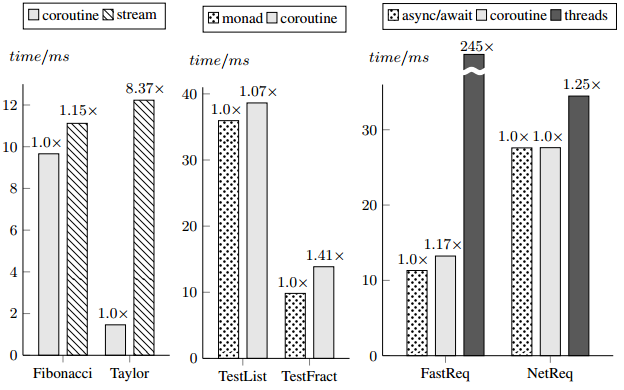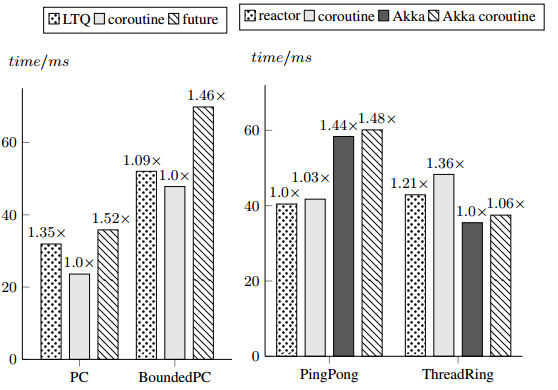# Scala Coroutines

### First-Class Type-Safe Coroutines in Scala### Behavior trees

A planning model in which an AI agent decides on the next action by traversing a special data structure called a behavior tree.

Every behavior tree is composed of two node types:

They are the leaf nodes of a behavior tree.

They execute a side-effect, and return running, success or failure status.They are the leaf nodes of a behavior tree.

They execute a side-effect, and return running, success or failure status.They are the leaf nodes of a behavior tree.

They execute a side-effect, and return running, success or failure status.### Control nodes

Control nodes are inner nodes that bind subtrees together.

Given a set of subtrees and their return values, a control node decides whether to run some subtree, or return a success or failure status.

Two basic control nodes: sequence and selector.### Behavior trees can compose

The main reason behind their popularity.Different subtrees can be developed, tuned and tested independently, and then merged into larger functional units.

### Behavior tree extensions

The basic model can be extended with nodes such as
repeaters, randomizers or inverters.### Behavior tree DSLs

```<?xml version="1.0" encoding="UTF-8"?> <agent> <selector> <sequence name="capture-the-flag> <task name="move"></task> <task name="take-the-flag"></task> </sequence> <module name="defend-the-base"> </module> </selector> </agent> ```

### IsomorphismBehavior tree planner is nothing more than an AST interpreter.### Behavior tree downsides

AI researchers have been rediscovering the wheel.

• implementing behavior trees is unintuitive
• nobody likes XML
• essentially an AST interpreter - bad performance
• harder to debug

### Why not just use a programming language?

``` def agent() = { captureTheFlag() || defendTheBase() } ``` ```def captureTheFlag(): Boolean = { move() && takeTheFlag() } ```

### Why not just use a programming language?

``` def agent() = { captureTheFlag() || defendTheBase() } ``` ```def captureTheFlag(): Boolean = { move() && takeTheFlag() } ```

Most languages cannot suspend computation and resume it later.

### Coroutines - the missing link

A programming construct that allows suspending the computation (i.e. yielding), and resuming it later from the point where it was suspended.

``` def captureTheFlag(): Boolean = { yield(move()) && takeTheFlag() } ```

### Goals of this talk

• Demonstrate how coroutines work in Scala
• Show how Scala coroutines generalize other approaches

### Subroutines

``` val double = (x: Int) => x + x ``` ``` double(7) ```

### Calling a subroutine```val double = (x: Int) => x + x double(7) ```

### Calling a subroutine```val double = (x: Int) => 7 + 7 double(7) ```

### Calling a subroutine```val double = (x: Int) => 14 double(7) ```

### Calling a subroutine```val double = ... 14 ```

### Calling a subroutineA function invocation is an entity that exists during program execution.

However, the callsite cannot observe the existence of that entity.

### Coroutine definition

``` val double = (x: Int) => x + x ```

### Coroutine definition

``` val double = coroutine { (x: Int) => x + x } ```

This coroutine does not yield.

The `yieldval` construct suspends computation
and yields a value to the caller.

### Coroutine definition

``` val double = coroutine { (x: Int) => yieldval(x) yieldval(x) } ```

### Calling a coroutine### Calling a coroutine### Calling a coroutine### Calling a coroutine### Calling a coroutine### Calling a coroutine### Calling a coroutine### Calling a coroutine### Calling a coroutineCoroutine invocation is resumed by the caller.
Therefore, it is an observable entity in the program.

### Calling a coroutine

``` val double = coroutine { (x: Int) => yieldval(x) yieldval(x) } ``` ```val i = call(double(7)) ```

A coroutine invocation can be observed by the callsite.

Therefore, it must be a first-class object.

### Calling a coroutine

``` val double = coroutine { (x: Int) => yieldval(x) yieldval(x) } ``` ```val i = call(double(7)) ``` ```var sum = 0 ``` ```while (i.resume) { sum += i.value } ```

### Calling a coroutine

``` val double = coroutine { (x: Int) => ↑yieldval(x) yieldval(x) } val i = call(double(7))↑ var sum = 0 while (i.resume) { sum += i.value } ```

### Calling a coroutine

``` val double = coroutine { (x: Int) => ↑yieldval(x) yieldval(x) } val i = call(double(7)) var sum = 0 while (i.resume↑) { sum += i.value } ```

### Calling a coroutine

``` val double = coroutine { (x: Int) => yieldval(x)↑ yieldval(x) } val i = call(double(7)) var sum = 0 while (i.resume↑) { sum += i.value } ```

### Calling a coroutine

``` val double = coroutine { (x: Int) => yieldval(x)↑ yieldval(x) } val i = call(double(7)) var sum = 0 while (i.resume) { sum += i.value↑ } ```

### Calling a coroutine

``` val double = coroutine { (x: Int) => yieldval(x)↑ yieldval(x) } val i = call(double(7)) var sum = 0 while (i.resume↑) { sum += i.value } ```

### Calling a coroutine

``` val double = coroutine { (x: Int) => yieldval(x) yieldval(x)↑ } val i = call(double(7)) var sum = 0 while (i.resume↑) { sum += i.value } ```

### Calling a coroutine

``` val double = coroutine { (x: Int) => yieldval(x) yieldval(x)↑ } val i = call(double(7)) var sum = 0 while (i.resume) { sum += i.value↑ } ```

### Calling a coroutine

``` val double = coroutine { (x: Int) => yieldval(x) yieldval(x)↑ } val i = call(double(7)) var sum = 0 while (i.resume↑) { sum += i.value } ```

### Calling a coroutine

``` val double = coroutine { (x: Int) => yieldval(x) yieldval(x) }↑ val i = call(double(7)) var sum = 0 while (i.resume↑) { sum += i.value } ```

### Calling a coroutine

``` val double = coroutine { (x: Int) => yieldval(x) yieldval(x) }↑ val i = call(double(7)) var sum = 0 while (i.resume) { sum += i.value } ↑ ```

### Coroutine instance operations

• `call` - creates a coroutine instance
• `resume` - resumes a coroutine instance
• `value` - obtains the last yielded value
• `result` - obtains the result of the invocation

### Typing a coroutine

``` val double = coroutine { (x: Int) => yieldval(x) yieldval(x) } ```

### Typing a coroutine

``` val double: Int ~> (Int, Unit) = coroutine { (x: Int) => yieldval(x) yieldval(x) } ``` ``` val i: Int <~> Unit = call(double(7)) ```

### Coroutine composition

As coroutine definitions grow larger,
so does the need to decompose them into independent components.

### Example: hash table

A closed-addressing hash table is an array containing buckets.
A bucket is a list of elements.

``` val array: Array[List[T]] ```

### Example: hash table

Assume that we know how to traverse a list of elements.

``` val bucket = coroutine { (b: List[T]) => ``` ``` var cur = b ``` ``` while (cur != Nil) { ``` ``` yieldval(cur.head) ``` ``` cur = cur.tail } } ```

### Example: hash table

Then, we should be able to use that to traverse an array of lists.

```val table = coroutine { (t: Array[List[T]]) => ``` ``` var i = 0 ``` ``` while (i < t.length) { ``` ``` bucket(t(i)) ``` ``` i += 1 } } ```

### Coroutine composition### Coroutine composition### Coroutine composition### Coroutine composition### Coroutine composition### Coroutine composition### Coroutine compositionThe direct call reuses the stack of the same coroutine instance.

### Use-cases

How do Scala coroutines generalize other models?

### Iterators

``` def iterator(tree: Tree) = call(foreach(tree)) ``` ``` val it = tree.iterator while (it.resume) println(it.value) ```

### Iterators

Iterators follow directly from `foreach` definitions.

``` val foreach = (t: Tree[T], f: T => Unit) => if (t != null) foreach(t.left, f) f(t.elem) foreach(t.right, f) } ```

### Iterators

Iterators follow directly from `foreach` definitions.

``` val foreach = coroutine { (t: Tree[T]) => if (t != null) foreach(t.left) yieldval(t.elem) foreach(t.right) } } ```

### Async-Await

```def loginRequest(): Future[String] def httpRequest(c: String): Future[Page] ``` ```async { val credential = await { loginRequest() } val ui = await { httpRequest(credential) } ui.html } ```

### Async-Await

How do we define `async` and `await` using coroutines?

### Async-Await

```def await[R]: Future[R] ~> (Future[R], R) = ``` ``` coroutine { (f: Future[R]) => ``` ``` yieldval(f) ``` ``` f.value.get.get } ```

### Async-Await

```def async[R](b: () ~> (Future[Any], R)) = { ``` ``` val i = call(b()) ``` ``` val p = Promise[R]() ``` ``` @tailrec def loop(): Unit = if (i.resume) ``` ``` i.value.onSuccess(loop) ``` ``` else p.success(i.result) ``` ``` Future { loop() } p.future } ```

### Direct-Style Reactors

An actor must declare all receive operations
in terms of a top-level `receive`.

``` class Printer extends Actor { def receive = { case x: Int => println(x) } } ```

### Direct-Style Reactors

A reactor uses first-class events sources,
and receives by calling `onEvent`.

``` class Printer extends Reactor[Int] { main.events.onEvent { x => println(x) } } ```

### Direct-Style Reactors

A reactor uses first-class events sources,
and receives by calling `onEvent`.

``` class Adder extends Reactor[Int] { operands.once.onEvent { x => operands.once.onEvent { y => println(x + y) } } } ```

### Direct-Style Reactors

This soon results in the pyramid of doom.
Instead, we want to write code without nesting.

``` class Adder extends Reactor[Int] { val x = operands.get() val y = operands.get() println(x + y) } ```

### Direct-Style Reactors

Challenge: implement methods `react` and `get`,
that define a reactor, and extract an event from its event source.

### Direct-Style Reactors

``` type Obs = (() => Unit) => Unit ``` ``` def get: () ~> (Obs, T) = ``` ``` coroutine { () => var ret: T = _ ``` ``` val obs = (cont: () => Unit) => onEvent(x => { ret = x; cont() }) ``` ``` yieldval(obs) ``` ``` ret } ```

### Direct-Style Reactors

``` type Obs = (() => Unit) => Unit ``` ``` def react[T](c: () ~> (Obs, Unit)) = ``` ``` Reactor[T] { val i = call(c()) ``` ``` def loop() = if (i.resume) i.value(loop) ``` ``` loop() } ```

### Simpler ScalaCheck tests

ScalaCheck tests typically used generators to explore the input space.

``` val tuples: Gen[(Int, Int)] = for { a <- choose(0, Int.MaxValue) b <- choose(0, a) } yield (a, b) ``` ``` property("comm") = forAll(tuples) { a + b == b + a } ```

### Simpler ScalaCheck tests

More intuitive: backtracking without inversion of control.

``` property("comm") = { val a = choose(0 until Int.MaxValue) val b = choose(0 until a) a + b == b + a } ```

### Simpler ScalaCheck tests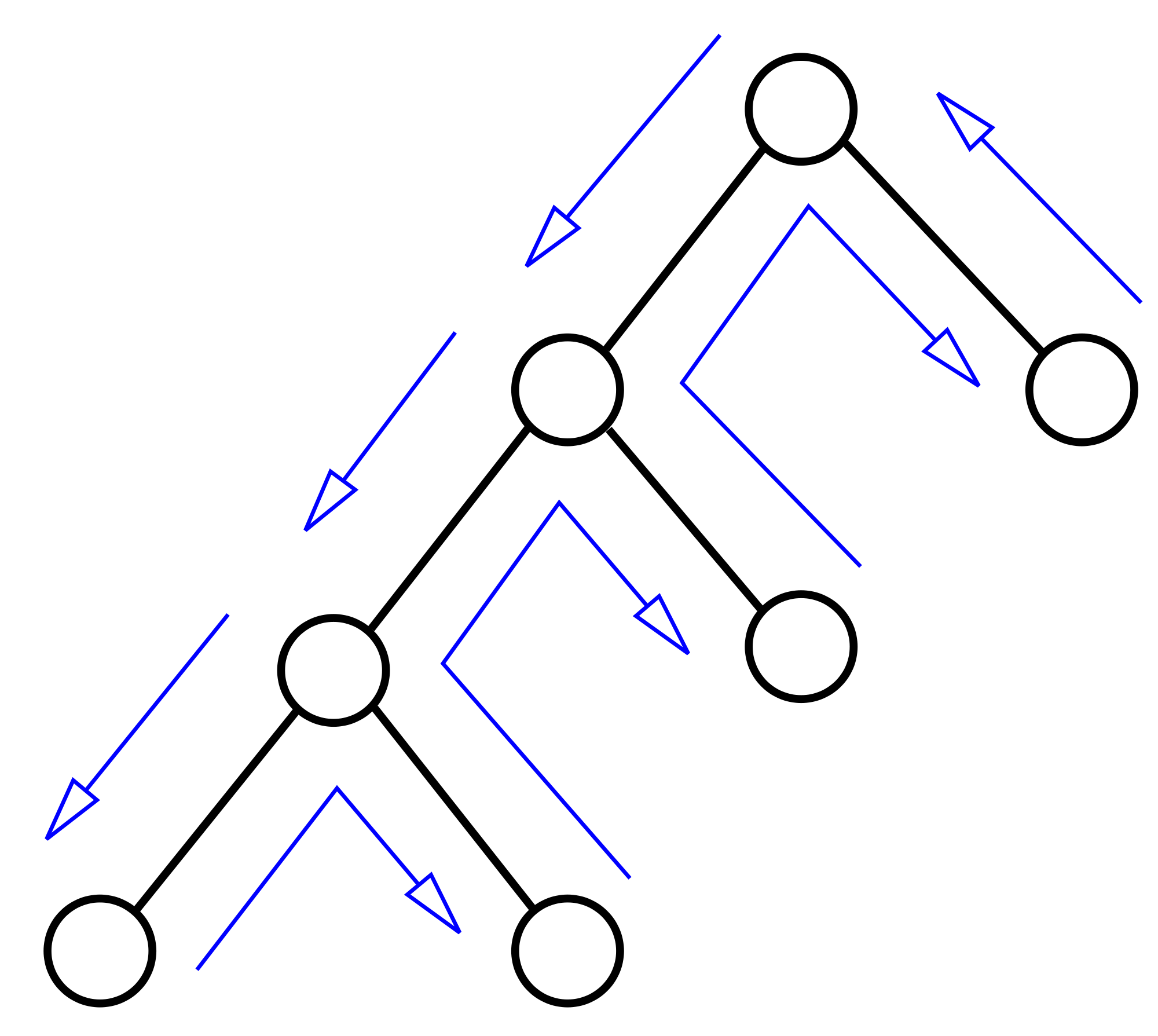### Simpler ScalaCheck tests

```type Program = Seq[() => Unit] <~> Unit ``` ``` val choose: Seq[Int] ~> (Seq[() => Unit], Int) = ``` ``` coroutine { (vals: Seq[Int]) => var res: Int = _ yieldval(vals.map(x => () => res = x)) ``` ``` res } ```

### Simpler ScalaCheck tests

```type Program = Seq[() => Unit] <~> Unit ``` ``` val backtrack: Program ~> (Unit, Unit) = coroutine { (p: Program) => ``` ``` if (p.resume) { ``` ``` for (prepare <- p.value) { prepare() ``` ``` backtrack(p.snapshot) } ``` ``` } else yieldval(()) } ```

### Simpler ScalaCheck tests

``` type Test = () ~> (Seq[() => Unit], Unit) ``` ``` val forever = coroutine { (test: Test) => while (true) { val p = call(test()) backtrack(p) } } ```

### Simpler ScalaCheck tests

``` def property(t: Test) = { val i = call(forever(t)) for (i <- 0 until MAX_TESTS) i.resume } ``` ``` property { val a = choose(0 until Int.MaxValue) val b = choose(0 until Int.MaxValue) a + b == b + a } ```

### Equivalence with delimited continuations

```type Shift = (() => Unit) => Unit def reset(b: () ~> (Shift, Unit)) = { def continue(i: Shift <~> Unit) = if (i.resume) i.value(() => continue(i.snapshot)) continue(call(b())) } def shift: Shift ~> (Shift, Unit) = coroutine { (b: Shift) => yieldval(b) } ```

### The most important type alias

``` type CoroutineAPI = ``` ``` CallbackStyleAPI => DirectStyleAPI ``` ``` implicit val aBitOfWork = implicitly[TwentyLinesOfCode] ```

## Thank you!http://github.com/storm-enroute/coroutines